Volume 65 Supplement 3

# Far-ultraviolet and far-infrared bivariate luminosity function of galaxies: Complex relation between stellar and dust emission

## Abstract

Far-ultraviolet (FUV) and far-infrared (FIR) luminosity functions (LFs) of galaxies show a strong evolution from z = 0 to z = 1, but the FIR LF evolves much stronger than the FUV one. The FUV is dominantly radiated from newly-formed short-lived OB stars, while the FIR is emitted by dust grains heated by the FUV radiation field. It is known that dust is always associated with star formation activity. Thus, both FUV and FIR are tightly related to the star formation in galaxies, but in a very complicated manner. In order to disentangle the relation between FUV and FIR emissions, we estimate the UV-IR bivariate LF (BLF) of galaxies with GALEX and AKARI All-Sky Survey datasets. Recently, we developed a new mathematical method to construct the BLF with given marginals and a prescribed correlation coefficient. This method makes use of a tool from mathematical statistics: the so-called “copula”. The copula enables us to construct a bivariate distribution function from given marginal distributions with a prescribed correlation and/or dependence structure. With this new formulation, and FUV and FIR univariate LFs, we analyze various FUV and FIR data with GALEX, Spitzer, and AKARI, to estimate the UV-IR BLF. The obtained BLFs naturally explain the nonlinear complicated relation between FUV and FIR emission from star-forming galaxies. Though the faint-end of the BLF was not well constrained for high-z samples, the estimated linear correlation coefficient ρ was found to be very high, and is remarkably stable regarding redshifts (from 0.95 at z = 0, to 0.85 at z = 1.0). This implies that the evolution of the UV-IR BLF is mainly due to the different evolution of the univariate LFs, and may not be controlled by the dependence structure.

## 1. Introduction

Exploring the star formation history of galaxies is one of the most important topics in modern observational cosmology. In particular, the “true” absolute value of the cosmic star formation rate (hereafter SFR) has been of central importance to an understanding of the formation and evolution of galaxies.

However, this has been a difficult task for a long time because of dust extinction. Active star formation (SF) is always accompanied by dust production through various dust grain formation processes related to the final stage of stellar evolution (e.g., Dwek and Scalo, 1980; Dwek, 1998; Nozawa et al., 2003; Takeuchi et al., 2005c; Asano et al., 2013). Observationally, the SFR of galaxies are, in principle, measured by the ultraviolet (UV) luminosity from massive stars because of their short lifetime (~106 yr) compared with the age of galaxies or the Universe. However, since the UV photons are easily scattered and absorbed by dust grains, the SFR of galaxies is always inevitably affected by dust produced by their own SF activity. Since the absorbed energy is re-emitted at wavelengths of the far-infrared (FIR), we need observations both in the UV and FIR to gain an unbiased view of their SF (e.g., Buat et al., 2005; Seibert et al., 2005; Takeuchi et al., 2005a, 2010; Cortese et al., 2006; Bothwell et al., 2011; Haines et al., 2011; Hao et al., 2011).

After much effort of many researchers, the cosmic history of the SFR density is gradually converging at 0 < z < 1. This “latter half” of the cosmic SFR is characterized by the rapid decline of the total SFR, especially the decrease of the contribution of dusty IR galaxies toward z = 0: While, at z = 0, the contribution of the SFR hidden by dust is 50–60%, it becomes >90% at z = 1 (Takeuchi et al., 2005a). This difference in the decrease in SFR obtained from FUV and FIR has already been recognized in IR studies (e.g., Takeuchi et al., 2001a, b). Later works have confirmed this “dusty era of the Universe”, and revealed that the dominance of the hidden SF continues even toward higher redshifts (z ~ 3) (e.g., Murphy et al., 2011; Cucciati et al., 2012).

Consequently, a natural question arises: what does the different evolution at different wavelengths represent? To address this problem, it is very important to understand how we select sample galaxies and what we see in them. Each time we find some relation between different properties, we must understand clearly which is real (physical) and which is simply due to a selection effect. Some are detected at both bands, but some are detected only at one of the observed wavelength and appear as upper limits at the other wavelength. In previous studies, it was often found that various claims were inconsistent with each other, mainly because they were not based on a well-controlled sample of FUV and FIR selected galaxies. Recently, thanks to new large surveys, some attempts to explore the SFR distribution of galaxies in a bivariate way have been made, through the “SFR function” (e.g., Buat et al., 2007, 2009; Bothwell et al., 2011; Haines et al., 2011). These works are based on the FUV and FIR LFs and their sum, but have not yet addressed their dependence on each other. To explore further the bivariate properties of the SF in galaxies, a suitable tool is the UV-IR bivariate luminosity function (BLF).

However, constructing a BLF from two-wavelength data is not a trivial task. When we have a complete flux-limited 1 Footnote 1 multiwavelength dataset, we can estimate a univariate luminosity function (LF) at each band, but what we want to know is the dependence structure between luminosities at different bands. Mathematically, this problem is rephrased as follows: can we (re)construct a multivariate probability density function (PDF) satisfying prescribed marginals? Although there is an infinite number of degrees of freedom to choose the original PDF, if we can model the dependence between variables, we can construct such a bivariate PDF. A statistical tool for this problem is the so-called “copula” (see Section 2 for the definition).

The copula has been extensively used in financial engineering, for instance, but until recently there have been very few applications to astrophysical problems (e.g., Benabed et al., 2009; Koen, 2009; Sato et al., 2010, 2011; Scherrer et al., 2010). Takeuchi (2010) introduced the copula to the estimation problem of a BLF. In this work, we apply a copula-based BLF estimation to the UV-IR two-wavelength dataset from z = 0 to z = 1, using data from IRAS, AKARI 2 Footnote 2, Spitzer 3 Footnote 3, and GALEX 4 Footnote 4.

The layout of this paper is as follows: We define a copula, in particular the Gaussian copula, and formulate the copula-based BLF in Section 2. We then describe our UV and IR data in Section 3. In Section 4, we first formulate the likelihood function for the BLF estimation. Then, we show the results, and discuss the possible interpretation of the evolution of the UV-IR BLF. Section 5 is devoted to the summary.

Throughout the paper, we will assume ΩM0 = 0.3, ΩΛ0 = 0.7 and H0 = 70 km s−1 Mpc−1. The luminosities are defined as νL ν and expressed in solar units assuming L = 3.83 × 1033 erg s−1.

## 2. The Bivariate Luminosity Function Based on the Copula

### 2.1 Copula: general definition

First, we briefly introduce a copula. A copula C(u1, u2) is defined as follows:(1)

where F1 (x1) and F2 (x2) are two univariate marginal cumulative distribution functions (DFs) and G(x1, x2) is a bivariate DF. We note that all bivariate DFs have this form and we can safely apply this method to any kind of bivariate DF estimation problem (Takeuchi, 2010). In various applications, we usually know the marginal DFs (or equivalently, the PDFs) from the data. The problem then reduces to a statistical estimation of a set of parameters to determine the shape of the copula C (u1, u2). In the form of the PDF,(2)

where f1 (x1) and f2 (x2) are the PDFs of F1 (x1) and F2 (x2), respectively. On the second line, c(u1, u2) is referred to as a differential copula.

### 2.2 Gaussian copula

Since the choice of copula is literally unlimited, we have to introduce a guidance principle. In many data analyses in physics, the most familiar measure of dependence might be the linear correlation coefficient ρ. Mathematically speaking, ρ depends not only on the dependence of two variables but also the marginal distributions, which is not an ideal property as a dependence measure. Even so, a copula having an explicit dependence on ρ would be convenient. In this work, we use a copula with this property: the Gaussian copula.

One of the natural candidates with ρ may be a copula related to a bivariate Gaussian DF (for other possibilities, see Takeuchi, 2010). The Gaussian copula has an explicit dependence on a linear correlation coefficient by its construction. Let(3)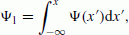(4)(5)

and(6)

where x ≡ (x1, x2)T, Σ is a covariance matrix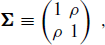(7)

and the superscript T stands for the transpose of a matrix or vector.

Then, we define a Gaussian copula CG(u1, u2; ρ) as(8)

The density of CG, cG, is obtained as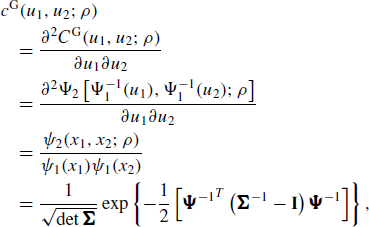(9)

where Ψ−1 ≡ [Ψ−1(u1), Ψ−1(u2)]T and I stands for the identity matrix.

### 2.3 Construction of the UV-IR BLF

In this work, we define the luminosity at a certain wavelength band by LνL ν (ν is the corresponding frequency). Then the LF is defined as the number density of galaxies whose luminosity lies between the logarithmic interval [log L, log L + d log L]: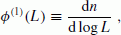(10)

where we denote log x ≡ log10x and ln x = log e x. For mathematical simplicity, we define the LF as being normalized, i.e.,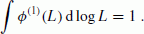(11)

Hence, this corresponds to a PDF. We also define a cumulative LF as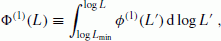(12)

where Lmin is the minimum luminosity of galaxies considered. This corresponds to the DF. If we denote the univariate LFs as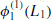and, then the BLF ϕ(2)(L1, L2) is described by a differential copula c(u1, u2) as(13)

For the Gaussian copula, the BLF is obtained as(14)

where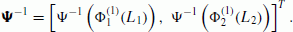(15)

For the UV LF, we adopt the Schechter function (Schechter, 1976):(16)

For the IR, we use the analytic form for the LF proposed by Saunders et al. (1990):(17)

We use the re-normalized version of Eqs. (17) and (16) so that they can be regarded as PDFs, as mentioned above.

### 2.4 Selection effects: another benefit of the copula BLF

Another benefit of the copula is that it is easy to incorporate observational selection effects which always exist in any kind of astronomical data. In a bi(multi)variate analysis, there are two categories of observational selection effects:

1. 1)

TruncationWe do not know if a source would exist below a detection limit.

2. 2)

CensoringWe know there is a source, but we have only an upper (sometimes lower) limit for a certain observable.

As mentioned above, we have to deal with both of these selection effects carefully to construct a BLF from the data at the same time. It is terribly difficult to incorporate these effects by heuristic methods: particularly for nonparamet-ric methods (Takeuchi, 2012, in preparation). In contrast, since we have an analytic form for the BLF, the treatment of upper limits is much more straightforward (Takeuchi, 2010). We show how it is treated in the likelihood function in Section 4.

## 3. Data

### 3.1 UV-IR data construction

We have constructed a dataset of galaxies selected at FUV and FIR by GALEX and IRAS for z = 0. At higher redshifts, GALEX and Spitzer data are used for z = 0.7 and EIS (ESO Imaging Survey) and Spitzer for z = 1.0 in the Chandra Deep Field South (CDFS). We explain the details of each sample in the following.

In the Local Universe, we used the sample compiled by Buat et al. (2007). This sample was constructed based on the IRAS all-sky survey and the GALEX All-Sky Imaging Survey (AIS). This dataset consists of UV- and IR-selected samples. The UV-selection was made by the GALEX FUV (λ = 1530 Å) band with FUV < 17 mag (hereafter, all magnitudes are presented in AB mag). This corresponds to the luminosity lower limit of LFUV > 108L. Red-shift information was taken from HyperLEDA (Paturel et al, 2003) and NED. The IR-selection is based on the IRAS PSCz (Saunders et al., 2000). The detection limit of the PSCz is S60 > 0.6 Jy. Redshifts of all PSCz galaxies were measured, and most of their redshifts are z < 0.05. All UV fluxes were remeasured with the package we have developed (Iglesias-Páramo et al., 2006) to avoid the shredding of galaxies. Details of the sample construction are explained in Buat et al. (2007). The number of the UV- and IR-selected samples are 606 and 644, respectively.

We also constructed a new, much larger sample of IR-selected galaxies by AKARI FIS All-Sky Survey. We started from the AKARI FIS bright source catalog (BSC) v.1 from the AKARI all sky survey (Yamamura et al., 2010). This sample is selected at the WIDE-S band (λ = 90 μ m) of the AKARI FIS (Kawada et al., 2007). The detection limit is S90 > 0.2 Jy. We first selected AKARI sources in the SDSS footprints to have the same solid angle with a (forthcoming) corresponding UV-selected sample we are preparing with GALEX-SDSS. 5 Footnote 5 Then, to have a secure sample of galaxies with redshift data, we made a cross-match of AKARI sources with the Imperial IRAS-FSC Redshift Catalogue (IIFSCz), a redshift catalog recently published (Wang and Rowan-Robinson, 2009), with a search radius of 36 arc-sec. Since about 90% of galaxies in the IIFSCz have spectroscopic, or photometric, redshifts at S60 > 0.36 Jy, the depth of the sample is defined by this matching. This determines the redshift range of this dataset to be approximately z < 0.1. We measured the FUV and NUV flux densities with the same procedure as the IRAS-GALEX sample. The detection limits at FUV and NUV of this sample are 19.9 mag and 20.8 mag (Morrissey et al., 2007). A corresponding UV-selected sample is under construction: hence, we only have an IR-selected sample. The number of galaxies is 3,891. For more information on this sample and the properties of galaxies, see Sakurai et al. (2013).

At higher-z, our samples are selected in the CDFS. GALEX observed this field at FUV and NUV (2300 Å) as a part of its deep imaging survey. We restricted the field to the subfield observed by Spitzer/MIPS, as a part of the GOODS key program (Elbaz et al., 2007), to have the corresponding IR data. The area of the region is 0.068 deg2. A precise description of our high-z samples is presented in Buat et al. (2009) and Burgarella et al. (2006).

At z = 0.7, the NUV-band corresponds to the rest-frame FUV of the sample galaxies. We thus constructed the sample based on NUV-selection. Redshifts were taken from the COMBO-17 survey (Wolf et al., 2004), and we have defined the z = 0.7 sample as those with redshifts of 0.6 < z < 0.8. Data reduction and redshift association are explained in Burgarella et al. (2006). We truncated the sample at NUV = 25.3 mag so that more than 90 % of the GALEX sources are identified in COMBO-17 with R < 24 mag. We set the MIPS 24 μ m upper limit as 0.025 mJy for undetected sources. For the IR-selected sample, we based on the GOODS Spitzer/MIPS 24 μ m sample and matched the GALEX and COMBO-17 sources. The sizes of the UV- and IR-selected samples are 340 and 470, respectively.

In contrast to z = 0.7, since NUV of GALEX corresponds to 1155 Å in the rest frame of galaxies at z = 1.0, we cannot use NUV as the primary selection band as the rest-frame FUV. Instead, we selected galaxies in the U-band from the EIS survey (Arnouts et al., 2001) that covers the CDFS/GOODS field. We then cross-matched the U-band sources with the COMBO-17 sample to obtain redshifts. For the z = 1 sample, the range of redshifts is 0.8 < z < 1.2. We set the limit at U = 24.3 mag to avoid source confusion. The IR flux densities were taken from Spitzer MIPS 24 μ m data, and the same upper limit as the z = 0.7 sample is taken for non-detections. Again, the IR-selected sample was constructed from the GOODS and matched the GALEX and COMBO-17 sources, as the z = 0.7 sample. The sizes of the z = 1.0 UV and IR-selected samples are 319 and 1033, respectively.

The characteristics of these samples are summarized in Table 1.

### 3.2 Far-UV and total IR luminosities

We are interested in the SF activity of galaxies and its evolution. Hence, luminosities of galaxies representative of SF activity would be ideal. As for the directly-visible SF, obviously the UV emission is appropriate for this purpose. We define the FUV luminosity of galaxies, LFUV, as LFUVνL ν @FUV. For z = 0 galaxies, FUV corresponds to 1530 Å. At higher redshifts, as we have explained, LFUV is calculated from the NUV flux density at z = 0.7 and the U-band flux density at z = 1.0, respectively.

In contrast, at IR, the luminosity related to the SF activity is the one integrated over a whole range of IR wavelengths (λ = 8–1000 μ m), L tir , where the subscript TIR stands for the total IR. For the Local IRAS-GALEX sample, it is quite straightforward to define Ltir since the IRAS galaxies are selected at 60 μ m. We adopted the formula L tir = 2.5νL ν @60 μ m. This rough approximation is, in fact, justified by the spectral energy distributions (SEDs) of galaxies with ISO 160 μ m observations (Takeuchi et al., 2006).

Also, for the AKARI-GALEX sample, we have excellent flux density data at FIR. We used the following TIR estimation formula from AKARI two widebands (Takeuchi et al., 2005b, 2010),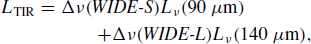(18)

where(19)

However, since deep FIR data of higher-z galaxies are not easily available to date, we should rely on the data taken by Spitzer MIPS 24 μ m. We use the conversion formulae from MIR luminosity to L tir :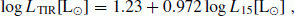(20)(21)

which are an updated version of the formulae proposed by Takeuchi et al. (2005b) and also used by Buat et al. (2009). Here, L15 and L12 are luminosities νL ν @15μm and 12 μ m, i.e., 24/(1 + z) at z = 0.7 and 1.0, respectively. The estimated L tir depends slightly on which kind of conversion formula is used; but for our current purpose, it does not affect our conclusion and we do not discuss this extensively here. An intercomparison of the MIR-TIR conversion formulae can be found in Buat et al. (2009).

This situation will be greatly improved with Herschel 6 Footnote 6

## 4. Results and Discussion

### 4.1 FUV and TIR univariate LFs

In order to estimate the UV-IR BLF, first we have to examine our setting for the FUV and TIR univariate LFs. The IRAS-GALEX sample, the validity of the Local univariate LFs are already proved (see figure 3 of Buat et al., 2007). Hence, we can safely use the analytic formulae of FUV and TIR LFs at z = 0 (Eqs. (16) and (17)).

We use the Schechter parameters presented by Wyder et al. (2005) for GALEX FUV: (α1, L1, ϕ1) = (1.21, 1.81 × 109h−2 [L], 1.35 × 10−2h3 [Mpc−3]). For the TIR, we used the parameters (α2, L2, ϕ2, σ) = (1.23, 4.34 × 108h−2 [L], 2.34 × 10−2h3 [Mpc−3], 0.724) (Takeuchi et al., 2003) obtained from the IRAS PSCz galaxies (Saunders et al., 2000), and multiplied L1 with 2.5 to convert the 60-μ m LF to the TIR LF.

For higher redshifts, ideally we should estimate the univariate FUV and TIR LFs simultaneously with the BLF estimation. This is, however, quite difficult with our current samples, because of the limited number of galaxies. We used, instead, the LF parameters at z = 0.7 and 1.0 obtained by previous studies on univariate LFs and modeled the FUV and TIR univariate LFs at these redshifts and examined their validity with nonparametric LFs estimated from the data. We use the parameters compiled by Takeuchi et al. (2005a).Parameters of the evolution of the TIR LF are obtained by approximating the evolution in the form(22)

where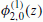is the local functional form of the TIR LF. Le Floc’h et al. (2005) assumed a power-law form for the evolution functions as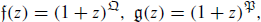(23)

and obtainedand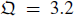, with α remaining constant. The Schechter parameters at z = 0.7 and 1.0 for the FUV LF are directly estimated by Arnouts et al. (2005) and we adopt their values (see Table 1 of Takeuchi et al., 2005a).

Then, we estimated the FUV and TIR LFs using the stepwise maximum likelihood method and the variant of the C-method from our CDFS multiwavelength data (for the estimation method, see, e.g., Takeuchi et al., 2000; Johnston, 2011, and references therein). The obtained univariate LFs at z = 0.7 and 1.0 are presented in Figs. 1 and 2. We also show the analytic model LFs in these figures. We note that a well-known large density enhancement locates in the CDFS at z = 0.7 (e.g., Salimbeni et al., 2009), and thus we have renormalized the LFs to remove the effect of the overden-sity. In the following analysis, we normalize the univariate LFs according to Eq. (11) so that we can treat the univariate LFs as PDFs; hence, this does not affect the following analysis at all.

Because of the small sample size, the LF shape does not perfectly agree with the supposed functional forms, but the nonparametric LFs are acceptably similar to the analytic functions both for the FUV and TIR at each redshift. We stress that the analytic functions are not the fit to the data, but estimated from other studies. This implies that the estimated evolutionary parameters of the LFs work generally well. Thus, we can use the higher-z univariate LFs as the marginal PDFs for the estimation of the UV-IR BLFs.

### 4.2 Copula likelihood for the BLF estimation

By using the estimated univariate FUV and TIR LFs as given marginals, we can estimate only one parameter, the linear correlation ρ by maximizing the likelihood function. The structure of a set of two-band selected data isi k = 1… n k . Here, jband (band: UV or IR) stands for the upper limit flag: jband = 0: detection and jband = − 1: upper limit. Another index, k, is the indicator of the selected band, i.e., k = 1 means a sample galaxy is selected at UV and k = − 1 means it is selected at IR. The likelihood function is as follows:(24)

whereis the probability for the i k th galaxy to be detected at both bands and to have luminosities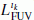and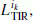,(25)is the probability for the i k th galaxy to be detected at the IR band and have a luminositybut not detected at the UV band and only an upper limitis available,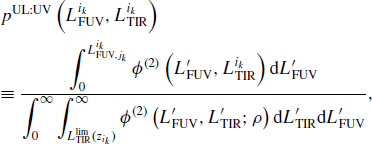(26)

and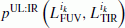is the probability for the i k th galaxy to be detected at the UV band and have a luminosity, but not detected at the IR band and only an upper limit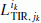is available,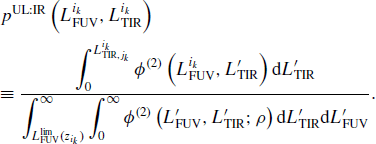(27)

The denominator in Eq. (25) is introduced to take into account the truncation in the data by observational flux selection limits at both bands (e.g., Sandage et al., 1979; Johnston, 2011). We should also note that it often happens that the same galaxies are included in both the UV- and IR-selected sample. In such a case, we should count the same galaxies only once to avoid double counting of them. Practically, such galaxies are included in any of the samples, because they are detected at both bands and are symmetric between UV- and IR-selections.

In the estimation procedure, we estimated the univariate LFs and their evolutions first, and then used these parameters to estimate the BLF and its evolution. One might wonder if this compromising method would introduce some bias in the estimation. Since we fix the form of the maximum likelihood estimator Eq. (24), this two-step estimation, instead of simultaneous estimation, does not bias the result, especially the dependence structure between UV and IR, unless the assumed univariate LF shape would be significantly different from the real one. We have already seen that the stepwise maximum likelihood estimation gave non-parametric LFs which agree reasonably with the assumed Schechter, or Saunders, function. Then, we can safely rely on the result for further discussion.

However, we should note that the two-step estimation gives conditional errors of the parameters only for each step, not the marginal ones. Then, the errors for each of the parameters are significantly underestimated. If we want to discuss the error values more precisely, we need a larger dataset and must use the simultaneous estimation method. This will be done in future work.

### 4.3 The BLF and its evolution

Using the Gaussian copula, we can now estimate the BLF. The visible and hidden SFRs should be directly reflected to this function. Dust is produced by SF activity, but also destroyed by SN blast waves as a result of the SF. Many physical processes are related to the evolution of the dust amount. Thus, first of all, we should describe statistically how it evolved, as stated in Introduction.

First, we summarize how to interpret the UV-IR BLF shown schematically in Fig. 3. First, we see the case that the ridge of the BLF is straight and diagonal (see (1) in Fig. 3). This means that the energy from SF is emitted equally at UV and IR with any SF activity. If the relation is diagonal, but has an offset horizontally or vertically, this suggests that a constant fraction of energy is absorbed by dust and re-emitted. Second, if the ridge is curved upward, it means that the more active the SF in a galaxy is, the more luminous at the IR (dusty SF: (2) in Fig. 3). Third, if the ridge is bent downward, the more active the SF is, the more luminous at UV (‘transparent’ SF: (3) in Fig. 3).

Now, we show the estimated BLFs in Figs. 47. In the Local Universe, the BLF is quite well constrained. The estimated correlation coefficient ρ is very high: ρ = 0.95 ± 0.04 for IRAS-GALEX and ρ = 0.95 ± 0.006 for AKARI-GALEX datasets. The apparent scatter of the L fuv L tir is found to be due to the nonlinear shape of the ridge of the BLF. This bent shape of the BLF was implied by preceding studies (Martin et al., 2005), and we have been able to quantify this feature. The copula BLF naturally reproduced it. For the AKARI-GALEX sample, only the IR-selected sample is available at this moment. This, however, does not bias the BLF estimation since the information from censored data points are incorporated properly in Eq. (24). This can be directly tested, for example, by using only one of the UV and IR-selected data for the z = 0 BLF estimation with the IRAS-GALEX dataset. Both one-band estimations with UV- and IR-selected data yield ρ = 0.95 ± 0.07. Obviously the error becomes larger because of fewer data, but the estimate itself remain unchanged.

At higher redshifts (z = 0.7−1.0), the linear correlation remains tight (ρ 0.91 ± 0.06 at z = 0.7 and ρ 0.86 ± 0.05 at z = 1.0), even though it is difficult to constrain the low-luminosity end from the data in this analysis (Spitzer-GALEX in the CDFS). The distribution of upper limits in Figs. 6 and 7 looks different from that in Fig. 4. Since we have restricted the redshifts of galaxies in these samples, the redshift restriction gives approximately constant luminosity limits both at FUV and FIR. This gives the “L-shaped” distribution of upper limits seen in these figures.

Though the whole shape cannot be perfectly determined by the current data, we find that ρ in the copula LF is high and remarkably stable regarding redshifts (from 0.95 at z = 0 to 0.85 at z = 1.0). This implies the evolution of the UV-IR bivariate LF is mainly due to the different evolution of the univariate LFs, and may not be controlled by the evolution of the dependence structure.

Forthcoming better data in the fauture will improve various aspects of the BLF estimation. First, Herschel, ALMA, and SPICA 7 Footnote 7

Second, when we have deeper data at UV and IR, we will be able to extend the luminosity range toward much lower luminosities both at FUV and FIR. Then, the faint-end structure of the BLF will be much more tightly determined. Further, it is not only for the determination of the faint end, but deeper data will also give some insights regarding the choice of the copula functional form. In this study, we did not try to examine whether the Gaussian copula would be a proper choice as a model of the UV-IR BLF. At z = 0, when UV-selected data as comparably large as the AKARI sample are available, we will be able to specify (a family of) copulas appropriate for this analysis. At higher redshifts, our current data are not deep enough to enable any conclusions on the faint-end dependence structure of the BLF, but, again, Herschel, ALMA, and SPICA data, together with ground-based optical or JWST data, will enable us to examine which copula would be appropriate to describe the BLF, and to constrain the evolution of the BLF along the whole history of galaxy evolution in the Universe.

## 5. Conclusion

To understand the visible and hidden SF history in the Universe, it is crucial to analyze multiwavelength data in a unified manner. The copula is an ideal tool to combine two marginal univariate LF to construct a bivariate LFs. It is straightforward to extend this method to multivariate DFs.

1. 1)

The Gaussian copula LF is sensitive to the linear correlation parameter ρ.

2. 2)

Even so, ρ in the copula LF is remarkably stable regarding redshifts (from 0.95 at z = 0 to 0.85 at z = 1.0).

3. 3)

This implies the evolution of the UV-IR BLF is mainly due to the different evolution of the univariate LFs, and may not be controlled by the dependence structure.

4. 4)

The nonlinear structure of the BLF is naturally reproduced by the Gaussian copula.

The data from Herschel, ALMA, and SPICA data will improve the estimates drastically, and we expect to specify the full evolution of the UV-IR BLF in the Universe. We stress that the copula will be a useful tool for any other kind of bi-(multi-) variate statistical analysis.

1. Or any other observational condition.

2. This step is not a mandatory in this study, but we are planning to make an extension of this analysis with the UV-selected data, and this step will make the analysis easier with respect to the treatment of survey areas when the UV-selected data will be ready.

3. URL: http://herschel.esac.esa.int/ data. We will leave this to our future work with the Herschel H-ATLAS (Eales et al., 2010) and GAMA (Galaxy And Mass Assembly: Driver et al., 2011) data (Takeuchi et al., 2012, in preparation).

4. URL: http://www.ir.isas.jaxa.jp/SPICA/SPICAHP/indexEnglish.html. will provide us with direct estimations of L TIR of galaxies, especially at high redshifts, since they will detect these galaxies at longer wavelengths than 24 μm. This allows us to avoid a possible bias of the estimated L TIR caused by the 24-μm-based extrapolation of the SED.

## References

• Arnouts, S. et al., ESO imaging survey. Deep public survey: Multi-color optical data for the Chandra Deep Field South, Astron. Astrophys., 379, 740–754, 2001.

• Arnouts, S. et al., The GALEX VIMOS-VLT Deep Survey Measurement of the Evolution of the 1500 A Luminosity Function, Astrophys. J., 619, L43–L46, 2005.

• Asano, R. S., T. T. Takeuchi, H. Hirashita, and A. K. Inoue, Dust formation history of galaxies: A critical role of metallicity for the dust mass growth by accreting materials in the interstellar medium, Earth Planets Space, 65, this issue, 213–222, 2013.

• Benabed, K., J.-F. Cardoso, S. Prunet, and E. Hivon, TEASING: a fast and accurate approximation for the low multipole likelihood of the cosmic microwave background temperature, Mon. Not. R. Astron. Soc, 400, 219–227, 2009.

• Bothwell, M. S., R. C. Kennicutt, B. D. Johnson, Y. Wu, J. C. Lee, D. Dale, C. Engelbracht, D. Calzetti, and E. Skillman, The star formation rate distribution function of the local Universe, Mon. Not. R. Astron. Soc, 415, 1815–1826, 2011.

• Buat, V. et al., Dust attenuation in the nearby universe: A comparison between galaxies selected in the ultraviolet and in the far-infrared, Astrophys. J., 619, L51–L54, 2005.

• Buat, V., T. T. Takeuchi, J. Iglesias-Páramo et al., The local universe as seen in the far-infrared and far-ultraviolet: A global point of view of the local recent star formation, Astrophys. J. Suppl. Ser, 469, 19–25, 2007.

• Buat, V., T. T. Takeuchi, D. Burgarella, E. Giovannoli, and K. L. Murata, The infrared emission of ultraviolet-selected galaxies from z = 0 to z = 1, Astron. Astrophys., 507, 693–704, 2009.

• Burgarella, D. et al., Ultraviolet-to-far infrared properties of Lyman break galaxies and luminous infrared galaxies at z ~ 1, Astron. Astrophys., 450, 69–76, 2006.

• Cortese, L., A. Boselli, V. Buat, G. Gavazzi, S. Boissier, A. Gil de Paz, M. Seibert, B. F. Madore, and D. C. Martin, UV dust attenuation in normal star-forming galaxies. I. Estimating the L tir /L fuv ratio, Astrophys. J., 637, 242–254, 2006.

• Cucciati, O. et al., The star formation rate density and dust attenuation evolution over 12 Gyr with the VVDS surveys, Astron. Astrophys., 539, A31, 2012.

• Driver, S. P. et al., Galaxy and Mass Assembly (GAMA): survey diagnostics and core data release, Mon. Not. R. Astron. Soc, 413, 971–995, 2011.

• Dwek, E., The evolution of the elemental abundances in the gas and dust phases of the galaxy, Astrophys. J., 501, 643, 1998.

• Dwek, E. and J. M. Scalo, The evolution of refractory interstellar grains in the solar neighborhood, Astrophys. J., 239, 193–211, 1980.

• Eales, S. et al., The Herschel ATLAS, Publ. Astron. Soc. Pac, 122, 499–515, 2010.

• Elbaz, D. et al., The reversal of the star formation-density relation in the distant universe, Astron. Astrophys., 468, 33–48, 2007.

• Haines, C. P., G. Busarello, P. Merluzzi, R. J. Smith, S. Raychaudhury, A. Mercurio, and G. P. Smith, ACCESS—II. A complete census of star formation in the Shapley supercluster—UV and IR luminosity functions, Mon. Not. R. Astron. Soc, 412, 127–144, 2011.

• Hao, C.-N., R. C. Kennicutt, B. D. Johnson, D. Calzetti, D. A. Dale, and J. Moustakas, Dust-corrected star formation rates of galaxies. II. Combinations of ultraviolet and infrared tracers, Astrophys. J., 741, 124 (22pp), 2011.

• Iglesias-Páramo, J. et al., Star formation in the nearby universe: The ultraviolet and infrared points of view, Astrophys. J. Suppl. Ser, 164, 38–51, 2006.

• Johnston, R., Shedding light on the galaxy luminosity function, Astron. Astrophys. Rev., 19, 41, 2011.

• Kawada, M. et al., The Far-Infrared Surveyor (FIS) for AKARI, Publ. Astron. Soc. Jpn., 59, 389–400, 2007.

• Koen, C, Confidence intervals for the correlation between the gamma-ray burst peak energy and the associated supernova peak brightness, Mon. Not. R. Astron. Soc, 393, 1370–1376, 2009.

• Le Floc’h, E. et al., Infrared luminosity functions from the Chandra Deep Field-South: The Spitzer view on the history of dusty star formation at 0 ≤ z ≤ 1, Astrophys. J., 632, 169–190, 2005.

• Martin, D. C. et al., The star formation rate function of the local universe, Astrophys. J., 619, L59–L62, 2005.

• Morrissey, P. et al., The calibration and data products of GALEX, Astron. Astrophys., 173, 682–697, 2007.

• Murphy, E. J., R.-R. Chary, M. Dickinson, A. Pope, and D. T. Frayer, An accounting of the dust-obscured star formation and accretion histories over the last ~ 11 billion years, Astrophys. J., 732, 126 (17pp), 2011.

• Nozawa, T, T. Kozasa, H. Umeda, K. Maeda, and K. Nomoto, Dust in the early universe: Dust formation in the ejecta of population III super-novae, Astrophys. J., 598,785–803, 2003.

• Paturel, G., C. Petit, P. Prugniel, G. Theureau, J. Rousseau, M. Brouty, P. Dubois, and L. Cambrésy, HYPERLEDA. I. Identification and designation of galaxies, Astron. Astrophys., 412, 45–55, 2003.

• Sakurai, A., T. T. Takeuchi, F.-T. Yuan, V. Buat, and D. Burgarella, Star formation and dust extinction properties of local galaxies as seen from AKARI and GALEX, Earth Planets Space, 65, this issue, 203–211, 2013.

• Salimbeni, S. et al., A comprehensive study of large-scale structures in the GOODS-SOUTH field up to z ~ 2.5, Astron. Astrophys., 501, 865–877, 2009.

• Sandage, A., G. A. Tammann, and A. Yahil, The velocity field of bright nearby galaxies. I—The variation of mean absolute magnitude with redshift for galaxies in a magnitude-limited sample, Astrophys. J., 232, 352–364, 1979.

• Sato, M., K. Ichiki, and T. T. Takeuchi, Precise estimation of cosmological parameters using a more accurate likelihood function, Phys. Rev. Lett., 105, 251301, 2010.

• Sato, M., K. Ichiki, and T. T. Takeuchi, Copula cosmology: Constructing a likelihood function, Phys. Rev. D, 83, 023501, 2011.

• Saunders, W., M. Rowan-Robinson, A. Lawrence, G. Efstathiou, N. Kaiser, R. S. Ellis, and C. S. Frenk, The 60-micron and far-infrared luminosity functions of IRAS galaxies, Mon. Not. R. Astron. Soc., 242, 318–337, 1990.

• Saunders, W. et al., The PSCz catalogue, Mon. Not. R. Astron. Soc., 317, 55–63, 2000.

• Schechter, P., An analytic expression for the luminosity function for galaxies, Astrophys. J., 203, 297–306, 1976.

• Scherrer, R. J., A. A. Berlind, Q. Mao, and C. K. McBride, From finance to cosmology: The copula of large-scale structure, Astrophys. J., 708, L9–L13, 2010.

• Seibert, M. et al., Testing the empirical relation between ultraviolet color and attenuation of galaxies, Astrophys. J., 619, L55–L58, 2005.

• Takeuchi, T. T., Constructing a bivariate distribution function with given marginals and correlation: application to the galaxy luminosity function, Mon. Not. R. Astron. Soc., 406, 1830–1840, 2010.

• Takeuchi, T. T., K. Yoshikawa, and T. T. Ishii, Tests of statistical methods for estimating galaxy luminosity function and applications to the Hubble deep field, Astrophys. J. Suppl. Ser., 129, 1–31, 2000.

• Takeuchi, T. T., T. T. Ishii, H. Hirashita, K. Yoshikawa, H. Matsuhara, K. Kawara, and H. Okuda, Exploring galaxy evolution from infrared number counts and cosmic infrared background, Publ. Astron. Soc. Jpn., 53, 37–52, 2001a.

• Takeuchi, T. T., R. Kawabe, K. Kohno, K. Nakanishi, T. T. Ishii, H. Hirashita, and K. Yoshikawa, Impact of future submillimeter and millimeter large facilities on the studies of galaxy formation and evolution, Publ. Astron. Soc. Pac., 113, 586–606, 2001b.

• Takeuchi, T. T., K. Yoshikawa, and T. T. Ishii, The luminosity function of IRAS point source catalog redshift survey galaxies, Astrophys. J., 587, L89–L92, 2003.

• Takeuchi, T. T., V. Buat, and D. Burgarella, The evolution of the ultraviolet and infrared luminosity densities in the universe at 0 < z < 1, Astron. Astrophys., 440, L17–L20, 2005a.

• Takeuchi, T. T., V. Buat, J. Iglesias-Páramo, A. Boselli, and D. Burgarella, Mid-infrared luminosity as an indicator of the total infrared luminosity of galaxies, Astron. Astrophys., 432, 423–429, 2005b.

• Takeuchi, T. T., T. T. Ishii, T. Nozawa, T. Kozasa, and H. Hirashita, A model for the infrared dust emission from forming galaxies, Mon. Not. R. Astron. Soc, 362, 592–608, 2005c.

• Takeuchi, T. T., T. T. Ishii, H. Dole, M. Dennefeld, G. Lagache, and J.-L. Puget, The ISO 170 µm luminosity function of galaxies, Astron. Astrophys., 448, 525–534, 2006.

• Takeuchi, T. T., V. Buat, S. Heinis, E. Giovannoli, F.-T. Yuan, J. Iglesias-Páramo, K. L. Murata, and D. Burgarella, Star formation and dust extinction properties of local galaxies from the AKARI-GALEX all-sky surveys. First results from the most secure multiband sample from the far-ultraviolet to the far-infrared, Astron. Astrophys., 514, A4, 2010.

• Wang, L. and M. Rowan-Robinson, The imperial IRAS-FSC redshift catalogue, Mon. Not. R. Astron. Soc, 398, 109–118, 2009.

• Wolf, C. et al., A catalogue of the Chandra Deep Field South with multicolour classification and photometric redshifts from COMBO-17, Astron. Astrophys., 421, 913–936, 2004.

• Wyder, T. K. et al., The ultraviolet galaxy luminosity function in the local universe from GALEX data, Astrophys. J., 619, L15–L18, 2005.

• Yamamura, I., S. Makiuti, N. Ikeda, Y. Fukuda, S. Oyabu, T. Koga, and G. J. White, AKARI/FIS all-sky survey bright source catalogue version 1.0 release note, ISAS/JAXA, 2010.

## Acknowledgments

This work is based on observations with AKARI, a JAXA project with the participation of ESA. This research has made use of the NASA/IPAC Extragalactic Database (NED) which is operated by the Jet Propulsion Laboratory, California Institute of Technology, under contract with the National Aeronautics and Space Administration. TTT has been supported by the Program for Improvement of Research Environment for Young Researchers from Special Coordination Funds for Promoting Science and Technology, and the Grant-in-Aid for the Scientific Research Fund (20740105, 23340046, 24111707) commissioned by the MEXT. TTT, AS, and FTY have been partially supported from the Grant-in-Aid for the Global COE Program “Quest for Fundamental Principles in the Universe: from Particles to the Solar System and the Cosmos” from the Ministry of Education, Culture, Sports, Science and Technology (MEXT) of Japan.

## Author information

Authors

### Corresponding author

Correspondence to Tsutomu T. Takeuchi.

## Rights and permissions

The images or other third party material in this article are included in the article’s Creative Commons licence, unless indicated otherwise in a credit line to the material. If material is not included in the article’s Creative Commons licence and your intended use is not permitted by statutory regulation or exceeds the permitted use, you will need to obtain permission directly from the copyright holder.

To view a copy of this licence, visit https://creativecommons.org/licenses/by/4.0/.

Reprints and Permissions

Takeuchi, T.T., Sakurai, A., Yuan, FT. et al. Far-ultraviolet and far-infrared bivariate luminosity function of galaxies: Complex relation between stellar and dust emission. Earth Planet Sp 65, 15 (2013). https://doi.org/10.5047/eps.2012.06.008

• Revised:

• Accepted:

• Published:

• DOI: https://doi.org/10.5047/eps.2012.06.008

### Key words

• Dust
• galaxies: formation
• galaxies: evolution
• stars: formation
• infrared
• ultraviolet